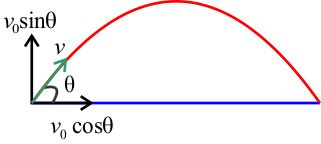# A projectile is shot from the ground at an angle of pi/4  and a speed of 3 /5 m/s. Factoring in both horizontal and vertical movement, what will the projectile's distance from the starting point be when it reaches its maximum height?

Feb 17, 2018

Consider,where theta = pi/4 = 45° in this case.

Moreover, recall that $\nu = 0$ at the maximum of the parabolic movement.

$\nu = {\nu}_{0} + a t$
$\implies t = \frac{\nu - {\nu}_{0}}{g}$

therefore t = (0 - (0.6m)/ssin(45°))/((-9.8m)/s^2) approx 4.32*10^-2s

Now: let's understand the horizontal displacement of the projectile. Recall,

$\Delta x = {\nu}_{0} \cos \theta \cdot t$

Hence,

Deltax = (0.6m)/scos(45°) * 4.32*10^-2s approx 2.60*10^-2m

This is fairly reasonable, $\frac{0.6 m}{s}$ is very slow.